# Due Monday, 11/01/1999

## 2.                  [1+1+2+2+2+2]         Unbound states of the finite square well

Consider the unbound states (E>0) of the finite square well. For a wave incident from the left, the solution to the Schrödinger equation can be written as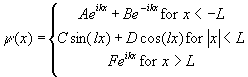.

a)      Determine k and l.

b)      Write down equations for all four boundary conditions for this problem.

c)      Use the boundary conditions at x=+L to express C in terms of F and to express D in terms of F.

d)      Use your results from c) and the boundary conditions at x=-L to eliminate A and to show that B can be expressed in terms of F as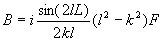with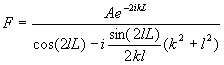.

e)      Plot the transmission coefficient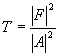versus energy over a suitable range.

f)        For which values of the energy is the transmission coefficient equal to 1?

## 3.                  [1+2+2+1+1]             Unbound states of the square potential barrier

Consider a particle with mass m and energy E>V0 incident from the left on a potential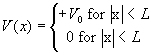.

a)      Write down the solution of Schrödinger’s equation for each region.

b)      State the boundary conditions that apply and obtain a sufficient number of equations to determine all unknown constants.

c)      Solve for the transmission coefficient and plot it versus energy over a suitable range.

d)      For which values of the energy is the transmission coefficient equal to 1?

e)      Which value does the transmission coefficient approach for E>>V0?